# Riesz decomposition theorem

(diff) ← Older revision | Latest revision (diff) | Newer revision → (diff)

There are two different theorems that go by this name.

## Riesz decomposition theorem for super- or subharmonic functions.

Roughly speaking, this asserts that a super- or subharmonic function is the sum of a potential and a harmonic function. For precise statements, see Subharmonic function (where it is called the Riesz local representation theorem), and Riesz theorem (where it is simply called the Riesz theorem), [a12], [a20]. See also [a8], 1.IV.8–9, 1.IX.11, 1.XIV.9, and [a8], 1.XV.7, 1.XVII.7, for a corresponding result for superparabolic functions. In [a4] the decomposition formula is called the Riesz integral representation and Riesz representation of a superharmonic function

There is also an abstract version (see also Potential theory, abstract), dealing with harmonic spaces, which states (see [a5], Thm. 2.2.2, p. 38) that every superharmonic functionon a harmonic space which has a subharmonic minorant may be written uniquely as the sum of a potential and a harmonic function. This harmonic function is the greatest hypo-harmonic minorant ofand is the infimum of any Perron set generated by.

An immediate consequence is the Brelot–Bauer theorem ([a5], Corol. 2.2.1, p. 38) that the real vector space of differences of positive harmonic functions on a harmonic space is a conditionally complete vector lattice (Riesz space) with respect to the natural order (i.e., pointwise comparison). This gives a link with the Riesz decomposition property.

There is also a converse Riesz decomposition theorem, [a11].

In the mid-1950s, the pioneering work of J.L. Doob and G.A. Hunt, [a7], [a14], [a15], [a16], showed a deep connection between potential theory and stochastic processes. Correspondingly, there are probabilistic Riesz decomposition theorems on decompositions of excessive functions, excessive measures and super-martingales. See [a3], [a9], [a8], 2.III.21, for precise formulations. There are also versions of these on commutative and non-commutative groups, [a1], [a2], [a6].

## Riesz decomposition theorem for operators.

This theorem is also called the Riesz splitting theorem and deals with splitting the spectrum of an operator. Following [a10], p. 9ff, letbe a bounded linear operator on a Banach spacewith spectrum. Letbe an isolated part of, i.e.andare both closed in. Let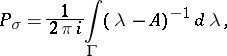whereis a contour in the resolvent set ofwithin its interior and separatingfrom. Then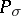is a projection (i.e.), called the Riesz projection or Riesz projector (cf. also Spectral synthesis (fora single point) and Krein space). Put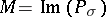,. Then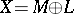, bothandare invariant under, and,.

If, moreover,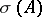is the disjoint union of two closed subsetsand, then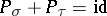,.

For more general results (for closed linear operators), see [a10], p. 326ff. See also Functional calculus (particularly the part dealing with the Riesz–Dunford functional calculus) and, e.g., [a13].

F. Riesz himself, to whom the original result is due, called it the Zerlegungssatz.

How to Cite This Entry:
Riesz decomposition theorem. Encyclopedia of Mathematics. URL: http://encyclopediaofmath.org/index.php?title=Riesz_decomposition_theorem&oldid=16314
This article was adapted from an original article by M. Hazewinkel (originator), which appeared in Encyclopedia of Mathematics - ISBN 1402006098. See original article